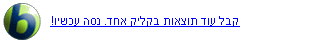English Wikipedia - The Free Encycl...
Electrical resistivity and conductivity
Electrical resistivity (also known as resistivity, specific electrical resistance, or volume resistivity) is an intrinsic property that quantifies how strongly a given material opposes the flow of electric current. A low resistivity indicates a material that readily allows the flow of electric current. Resistivity is commonly represented by the Greek letter ρ (rho). The SI unit of electrical resistivity is the ohmmetre (Ω⋅m) although other units like ohmcentimetre (Ω⋅cm) are also in use. As an example, if a solid cube of material has sheet contacts on two opposite faces, and the resistance between these contacts is 1 Ω, then the resistivity of the material is 1 Ω⋅m.See more at Wikipedia.org...